Click to Chat

1800-1023-196

+91-120-4616500

CART 0

• 0

MY CART (5)

Use Coupon: CART20 and get 20% off on all online Study Material

ITEM
DETAILS
MRP
DISCOUNT
FINAL PRICE
Total Price: Rs.

There are no items in this cart.
Continue Shopping• Complete JEE Main/Advanced Course and Test Series
• OFFERED PRICE: Rs. 15,900
• View Details

```Data Handling III (Pictorial Representation of Data as Pie Charts or Circle Graphs) Exercise 25.1

Question: 1

The number of hours, spent by a school boy on different activities in a working day, is given below:

Activities
Sleep
School
Home
Play
Others
Total

Number of hours
8
7
4
2
3
24

Present the information in the form of a pie-chart.

Solution:

We know:

Central angle of a component = (component value / sum of component values × 360) Here, total number of hours = 24

Thus, the central angle for each component can be calculated as follows:

Activity
Number of hours
Sector angle

Sleep
8
8/24 × 360 = 120°

School
7
7/24 × 360 = 105°

Home
4
4/24 × 360 = 60°

Play
2
2/24 × 360 = 30°

Others
3
3/24 × 360 = 45°\

Now, the pie chat that represents the given data can be constructed by following the steps given below:

Step 1: Draw a circle of an appropriate radius.

Step 2: Draw a vertical radius of the circle drawn in step 1.

Step 3: Choose the largest central angle. Here, the largest central angle is 120°. Draw a sector with the central angle 120° in such a way that one of its radii coincides with the radius drawn in step 2 and another

radius is in its counter-clockwise direction.

Step 4: Construct other sectors representing other items in the clockwise direction in descending order of magnitudes of their central angles.

Step 5: Shade the sectors with different colours and label them as shown as in the ﬁgure below.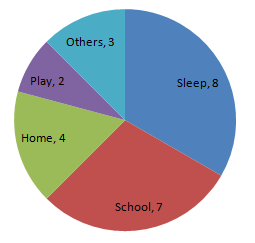Question: 2

Employees of a company have been categorized according to their religions as give

Religions
Hindu
Muslim
Sikh
Christian
Total

Number of workers
420
300
22
10
108

Draw a pie-chart to represent the above information.

Solution:

We know:

Central angle of a component = (component value / sum of component values     3

Here, total number of workers = 1050

Thus, the central angle for each component can be calculated as follows: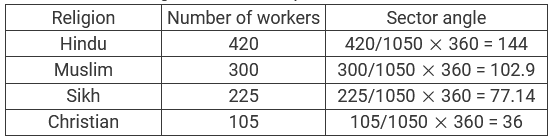Note: The total number of workers is 1050, not 1080.

Now, the pie chat that represents the given data can be constructed by following the steps below:

Step 1 : Draw circle of an appropriate radius.

Step 2 : Draw a vertical radius of the circle drawn in step 1.

Step 3 : Choose the largest central angle. Here, the largest central angle is 144o. Draw a sector with the central angle 144o in such a way that one of its radii coincides with the radius drawn in step 2 and another

radius is in its counter clockwise direction.

Step 4 : Construct other sectors representing other items in the clockwise direction in the descending order of magnitudes of their central angles.

Step 5 : Shade the sectors with different colours and label them as shown as in the ﬁgure below.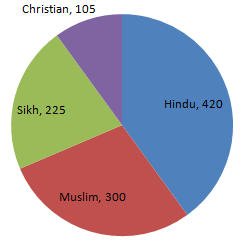Question: 3

In one day the sales (in rupees) of different items of a baker's shop are given below:

Items
Cakes and Pastries
Biscuits
Others
Total

Sales (in Rs)
260
40
100
60
20
480

Draw a pie-chart representing the above sales.

Solution: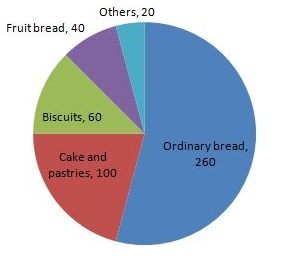Question: 4

The following data shows the expenditure of a person on different items during a month. Represent the data by a pie-chart.

Items of expenditure
Rent
Education
Food
Clothing
Others

Amount (in Rs)
2700
1800
2400
1500
2400

Solution: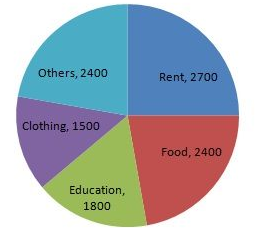Question: 5

The percentages of various categories of workers in a state are given in the following table.

Categoies
Culti-vators
Agricultural Labourers
Industrial Workers
Commercial Workers
Others

% of workers
40
25
12.5
10
12.5

Present the information in the form a pie-chart.

Solution:Question: 6

The following table shows the expenditure incurred by a publisher in publishing a book:

Items
Paper
Printing
Binding
Miscellaneous

Expenditure (in%)
35%
20%
10%
5%
30%

Present the above data in the form of a pie-chart.

Solution: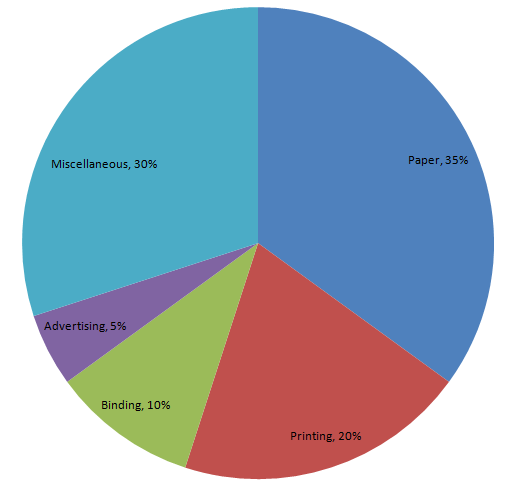Question: 7

Percentage of the different products of a village in a particular district are given below. Draw a pie-chart representing this information.Solution: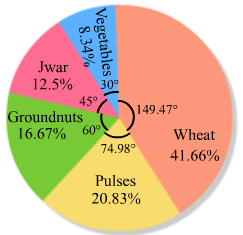```### Course Features

• 728 Video Lectures
• Revision Notes
• Previous Year Papers
• Mind Map
• Study Planner
• NCERT Solutions
• Discussion Forum
• Test paper with Video Solution